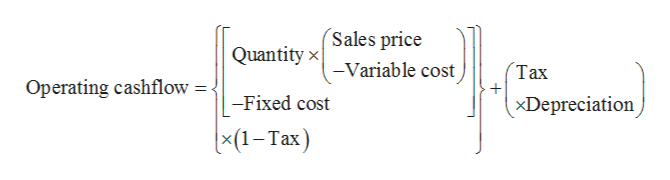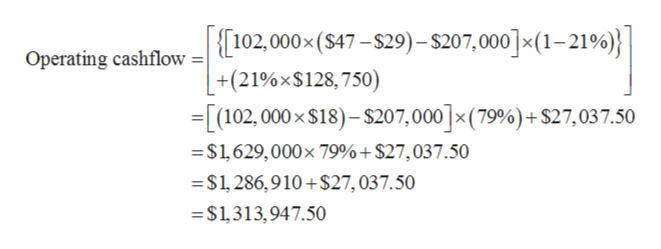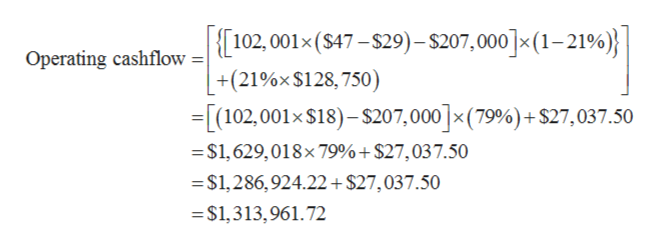# Consider a project with the following information: Initial fixed asset investment = \$515,000; straight-line depreciation to zero over the 4-year life; zero salvage value; price = \$47; variable costs = \$29; fixed costs = \$207,000; quantity sold = 102,000 units; tax rate = 21 percent.  How sensitive is OCF to changes in quantity sold? (Do not round intermediate calculations and round your answer to 2 decimal places, e.g., 32.16.)find the change in OCF/Change in Q

Question
3 views
 Consider a project with the following information: Initial fixed asset investment = \$515,000; straight-line depreciation to zero over the 4-year life; zero salvage value; price = \$47; variable costs = \$29; fixed costs = \$207,000; quantity sold = 102,000 units; tax rate = 21 percent.

 How sensitive is OCF to changes in quantity sold? (Do not round intermediate calculations and round your answer to 2 decimal places, e.g., 32.16.)

find the change in OCF/Change in Q

check_circle

Step 1

The initial fixed investment cost is \$515,000. So, annual depreciation will be (\$515,000 ÷ 4) = \$128,750.

The operating cashflow can be calculated with the help of below expression:help_outlineImage TranscriptioncloseSales price Quantity x -Variable cost Таx Operating cashflow= - xDepreciation -Fixed cost (x(1-Тах) fullscreen
Step 2

Substitute 102,000 for quantity, \$47 for sales price, \$29 for variable cost, \$207,000 for fixed cost, 21% for tax and \$128,750 for depreciation in the above expression,help_outlineImage Transcriptionclose[102,000x (S47-\$29)- \$207,000] x (1- 219%); Operating cashflow +(21%x\$128,750) -[(102, 000x \$18)-\$207,000] x (79%) + \$27,03 7.50 \$1,629,000x 79%+ \$27,037.50 \$1, 286,910+\$27, 037.50 \$1,313,947.50 fullscreen
Step 3

If quantity sold is increase by 1 unit, th...help_outlineImage Transcriptionclose102,001x (S47-\$29)- \$207,000]x (1-219%)| Operating cashflow +(21%x\$128,750) -[(102,001x \$18)-\$207,000]x (79%) +S27,037.50 \$1,629,018x79%+ \$27,037.50 \$1,286,924.22+ \$27,037.50 \$1,313,961.72 fullscreen

### Want to see the full answer?

See Solution

#### Want to see this answer and more?

Solutions are written by subject experts who are available 24/7. Questions are typically answered within 1 hour.*

See Solution
*Response times may vary by subject and question.
Tagged in